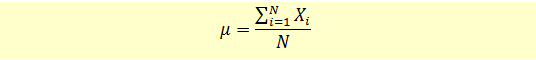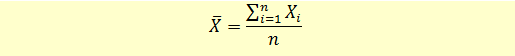# Calculating Arithmetic Mean

Arithmetic mean is the simple average of all observations and is calculated by adding all the observations and dividing it by the total number of observations.

We can calculate arithmetic mean for both the population and the sample.

Population Mean

This is the arithmetic mean of all observations in the population. The formula for population mean is given below:Sample Mean

This is the arithmetic mean of all observations in the sample of the population. The formula for sample mean is given below:Notice the difference in notations between the two formulas:

Xi represents the observations in both formulas.